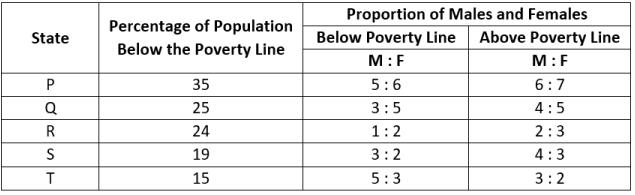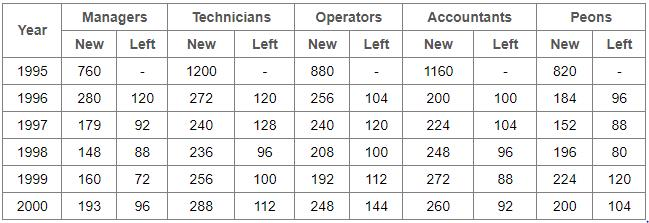Courses

# Practice Test: Table Charts - 1

## 5 Questions MCQ Test Logical Reasoning (LR) and Data Interpretation (DI) | Practice Test: Table Charts - 1

Description
This mock test of Practice Test: Table Charts - 1 for UPSC helps you for every UPSC entrance exam. This contains 5 Multiple Choice Questions for UPSC Practice Test: Table Charts - 1 (mcq) to study with solutions a complete question bank. The solved questions answers in this Practice Test: Table Charts - 1 quiz give you a good mix of easy questions and tough questions. UPSC students definitely take this Practice Test: Table Charts - 1 exercise for a better result in the exam. You can find other Practice Test: Table Charts - 1 extra questions, long questions & short questions for UPSC on EduRev as well by searching above.
QUESTION: 1

### The following table gives the percentage distribution of the population of five states, P, Q, R, S and T on the basis of the poverty line and also on the basis of sex.Q. If the male population above the poverty line for State R is 1.9 million, then the total population of State R is?

Solution:

Let the total population of state R be x million.
Then, the population of state R above poverty line is:

= [(100 - 24)% of x] million
= (76 / 100) * x million

So male populaion of state R above poverty line is:

= [2 / 5 * (76 / 100) * x] million

But, it is given that male population of state R above poverty line = 1.9 million.
∴ (2 / 5) * (76 / 100) * x) = 1.9
⇒ x = 5 * 100 * 1.9 / (76 * 2) = 6.25
∴ Total population of state R = 6.25 million.

QUESTION: 2

### The following table gives the percentage distribution of the population of five states, P, Q, R, S and T on the basis of the poverty line and also on the basis of sex.Q. What will be the number of females above the poverty line in the state S if it is known that the population of State S is 7 million?

Solution:

Total population of state S = 7 million
Population above poverty line is:

= [(100 - 19)% of 7] million
= (81% of 7) million
= 5.67 million

So the number of females above the poverty line in the state of S is:

= (3 / 7) * 5.67 million
= 2.43 million

QUESTION: 3

### The following table gives the percentage distribution of the population of five states, P, Q, R, S and T on the basis of the poverty line and also on the basis of sex.Q. What will be the male population above the poverty line for State P if the female population below the poverty line for State P is 2.1 million?

Solution:

Female population below poverty line for state P = 2.1 million
Let the male population below poverty line for state P be 'x' million.

5 : 6 = x : 21
x = 2.1 * (5 / 6) = 1.75

∴ Population below poverty line for state P = (2.1 + 1.75) million = 3.85 million
Let the population above poverty line for state P be 'y' million
Since, 35% of the total population of state P is below poverty line.
Therefore, 65% of the total population of state P is above poverty line i.e., the ratio of population below poverty line to that above poverty line of state P is 35 : 65

35 : 65 = 3.85 : y
y = 65 * 3.85 / 35 = 7.15

∴ Population above poverty line for state P = 7.15 million and so, male population above poverty line for state P is:

= (6 / 13) * 7.15 million
= 3.3 million

QUESTION: 4

The following table gives the percentage distribution of the population of five states, P, Q, R, S and T on the basis of the poverty line and also on the basis of sex.Q. If the population of males below the poverty line for State Q is 2.4 million and that for State T is 6 million, then the total populations of States Q and T are in the ratio?

Solution:

For state Q:
Male population below poverty line = 2.4 million
Let the female population below the poverty line be 'x' million.

3 : 5 = 2.4 : x
x = 5 * 2.4 / 3 = 4

∴ Total population below poverty line = (2.4 + 4) = 6.4 million
If Nq = 6.4 million ⇒ Nq = (6.4 x 100 / 25) million = 25.6 million
For state T:
Male population below poverty line = 6 million
Let the female population below the poverty line be 'y' million.

3 : 5 = 6 : y
y = 3 * 6 / 5 = 3.6

∴ Total population below poverty line = (2.4 + 4) = 6.4 million
If Nt = 6.4 million ⇒ Nt = (9.6 x 100 / 15) million = 64 million
Required ratio is:

= Nq / Nt = 25.6 / 64 = 0.4 = 2 / 5

QUESTION: 5

The following table shows the number of new employees added to different categories of employees in a company and also the number of employees from these categories who left the company every year since the foundation of the Company in 1995.Q. What is the difference between the total number of Technicians added to the Company and the total number of Accountants added to the Company during the years 1996 to 2000?

Solution:

Required difference is:

= (272 + 240 + 236 + 256 + 288) - (200 + 224 + 248 + 272 + 260)
= 88